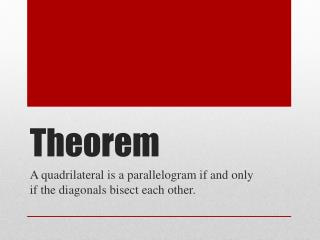DownloadDownload PresentationTheorem

# Theorem

Télécharger la présentation## Theorem

- - - - - - - - - - - - - - - - - - - - - - - - - - - E N D - - - - - - - - - - - - - - - - - - - - - - - - - - -
##### Presentation Transcript

1. Theorem A quadrilateral is a parallelogram if and only if the diagonals bisect each other.

2. The diagonals bisect each other. Line segment DE is congruent to line segment EB & line segment AE is congruent to line segment EC. What am I trying to prove?

3. ABCD is a parallelogram What is the given information?

4. I have triangles. If I can prove that triangle ABE and triangle DEC are congruent then I can show that line segment DE is congruent to line segment EB & line segment AE is congruent to line segment EC because of CPCTC. First I have to show that the two triangles are congruent. What do I know?

5. Since I know that ABCD is a parallelogram then I can say that AB is parallel to DC. I can use the theorem about parallel lines. How do I do that?

6. I can say that these two triangles are congruent because of ASA. I showed all of the requirements. Because I showed that triangle ABE and triangle DEC are congruent, I can state that DE is congruent to EB & AE is congruent to EC. Thus the two diagonal bisect each other. Conclusion